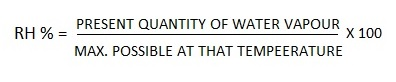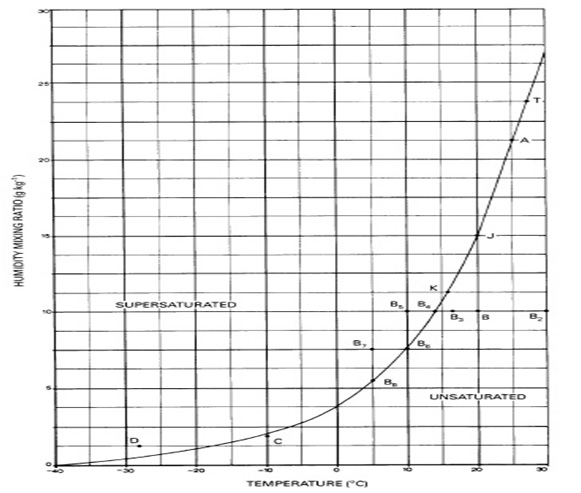# What is Humidity?

Humidity is the amount of water vapour present in the atmosphere.

Humidity Mixing Ratio- The ratio of the mass of water vapour to the mass of dry air (air without water vapour). Units are grams per kilogram (g kg- I).

Absolute Humidity is the mass of water vapour contained in the sample of air. This ratio is also known as the vapour density or vapour concentration.

Vapour Pressure- The pressure exerted by the water vapour in the atmosphere, which forms partof the total atmospheric pressure. Units are hectopascals (hPa).As its temperature increases, air has the capacity to hold more water vapour. The saturation curve shows the maximum amount which can be present at any given temperature, assuming that the saturated mass of air coexists in equilibrium with a plane liquid water surface. In above, the units of the vertical axis may be humidity mixing ratio, absolute humidity or vapour pressure.

Air is termed saturated when it contains the maximum amount of water vapour possible at a given temperature. In above figure, the mass of air (A) with a temperature of 25°C and a humidity mixing ratio of 21.25 g/kg is saturated. In this case the humidity mixing ratio is termed the saturated humidity mixing ratio. Unsaturated air, (B and C) contains less than the maximum amount of water vapour possible at its given temperature. Air is supersaturated (D) when it contains more water vapour than is needed to saturate it at a given temperature.